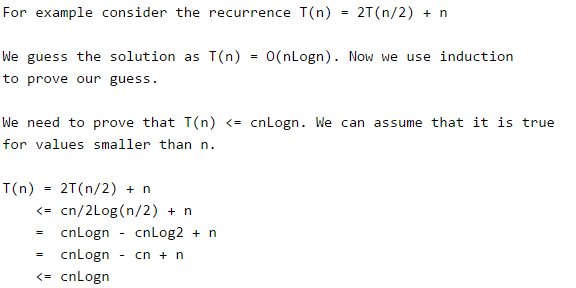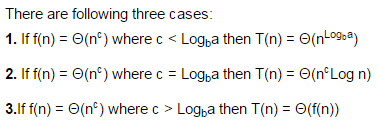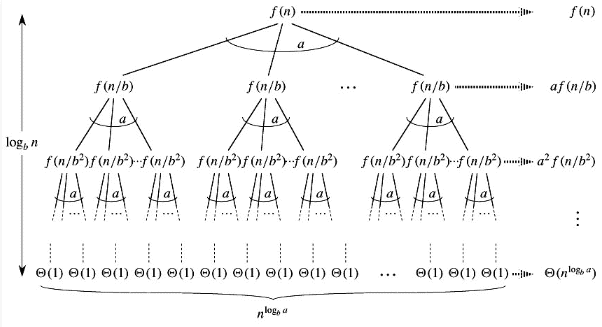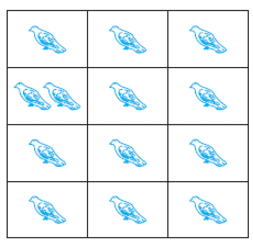GATE  >  Combinatorics

# Combinatorics Notes | Study GATE Computer Science Engineering(CSE) 2023 Mock Test Series - GATE

## Document Description: Combinatorics for GATE 2022 is part of Discrete Mathematics for GATE Computer Science Engineering(CSE) 2023 Mock Test Series preparation. The notes and questions for Combinatorics have been prepared according to the GATE exam syllabus. Information about Combinatorics covers topics like and Combinatorics Example, for GATE 2022 Exam. Find important definitions, questions, notes, meanings, examples, exercises and tests below for Combinatorics.

Introduction of Combinatorics in English is available as part of our GATE Computer Science Engineering(CSE) 2023 Mock Test Series for GATE & Combinatorics in Hindi for GATE Computer Science Engineering(CSE) 2023 Mock Test Series course. Download more important topics related with Discrete Mathematics , notes, lectures and mock test series for GATE Exam by signing up for free. GATE: Combinatorics Notes | Study GATE Computer Science Engineering(CSE) 2023 Mock Test Series - GATE
 1 Crore+ students have signed up on EduRev. Have you?

## Analysis of Algorithm | (Solving Recurrences)

Many algorithms are recursive in nature. When we analyze them, we get a recurrence relation for time complexity. We get running time on an input of size n as a function of n and the running time on inputs of smaller sizes. For example in Merge Sort, to sort a given array, we divide it in two halves and recursively repeat the process for the two halves. Finally we merge the results. Time complexity of Merge Sort can be written as T(n) = 2T(n/2) + cn. There are many other algorithms like Binary Search, Tower of Hanoi, etc.

There are mainly three ways for solving recurrences.

1) Substitution MethodWe make a guess for the solution and then we use mathematical induction to prove the the guess is correct or incorrect.2) Recurrence Tree Method: In this method, we draw a recurrence tree and calculate the time taken by every level of tree. Finally, we sum the work done at all levels. To draw the recurrence tree, we start from the given recurrence and keep drawing till we find a pattern among levels. The pattern is typically a arithmetic or geometric series.To know the value of T(n), we need to calculate sum of tree nodes level by level. If we sum the above tree level by level, we get the following series T(n) = c(n^2 + 5(n^2)/16 + 25(n^2)/256) + ....
The above series is geometrical progression with ratio 5/16. To get an upper bound, we can sum the infinite series. We get the sum as (n2)/(1 - 5/16) which is O(n2)

3) Master Method:
Master Method is a direct way to get the solution. The master method works only for following type of recurrences or for recurrences that can be transformed to following type.

`T(n) = aT(n/b) + f(n) where a >= 1 and b > 1`How does this work?
Master method is mainly derived from recurrence tree method. If we draw recurrence tree of T(n) = aT(n/b) + f(n), we can see that the work done at root is f(n) and work done at all leaves is Θ(nc) where c is Logba. And the height of recurrence tree is LogbnIn recurrence tree method, we calculate total work done. If the work done at leaves is polynomially more, then leaves are the dominant part, and our result becomes the work done at leaves (Case 1). If work done at leaves and root is asymptotically same, then our result becomes height multiplied by work done at any level (Case 2). If work done at root is asymptotically more, then our result becomes work done at root (Case 3).

Examples of some standard algorithms whose time complexity can be evaluated using Master Method
Mwrge Sort :T(n) = 2T(n/2) + Θ(n). It falls in case 2 as c is 1 and Logba] is also 1. So the solution is Θ(n Logn)

Binary Search:T(n) = T(n/2) + Θ(1). It also falls in case 2 as c is 0 and Logba is also 0. So the solution is Θ(Logn)

Notes:
1) It is not necessary that a recurrence of the form T(n) = aT(n/b) + f(n) can be solved using Master Theorem. The given three cases have some gaps between them. For example, the recurrence T(n) = 2T(n/2) + n/Logn cannot be solved using master method.

2) Case 2 can be extended for f(n) = Θ(ncLogkn)
If f(n) = Θ(ncLogkn) for some constant k >= 0 and c = Logba, then T(n) = Θ(ncLogk+1n)

## Discrete Mathematics | The Pigeonhole Principle

Suppose that a flock of 20 pigeons flies into a set of 19 pigeonholes to roost. Because there are
20 pigeons but only 19 pigeonholes, a least one of these 19 pigeonholes must have at least two
pigeons in it. To see why this is true, note that if each pigeonhole had at most one pigeon in it,
at most 19 pigeons, one per hole, could be accommodated. This illustrates a general principle
called the pigeonhole principle, which states that if there are more pigeons than pigeonholes,
then there must be at least one pigeonhole with at least two pigeons in it.Theorem:

I) If “A” is the average number of pigeons per hole, where A is not an integer then

• At least one pigeon hole contains ceil[A] (smallest integer greater than or equal to A) pigeons
• Remaining pigeon holes contains at most floor[A] (largest integer less than or equal to A) pigeons

Or

II) We can say as, if n + 1 objects are put into n boxes, then at least one box contains two or more objects.
The abstract formulation of the principle: Let X and Y be finite sets and let f: X –> Y be a function.

• If X has more elements than Y, then f is not one-to-one.
• If X and Y have the same number of elements and f is onto, then f is one-to-one.
• If X and Y have the same number of elements and f is one-to-one, then f is onto.

Pigeonhole principle is one of the simplest but most useful ideas in mathematics. We will see more applications that proof of this theorem.

Example 1: If (Kn+1) pigeons are kept in n pigeon holes where K is a positive integer, what is the average no. of pigeons per pigeon hole?

Solution: average number of pigeons per hole = (Kn+1)/n
= K + 1/n
Therefore at least a pigeonholes contains (K+1) pigeons i.e., ceil[K +1/n] and remaining contain at most K i.e., floor[k+1/n] pigeons.
i.e., the minimum number of pigeons required to ensure that at least one pigeon hole contains (K+1) pigeons is (Kn+1).

Example 2: A bag contains 10 red marbles, 10 white marbles, and 10 blue marbles. What is the minimum no. of marbles you have to choose randomly from the bag to ensure that we get 4 marbles of same color?
Solution: Apply pigeonhole principle.
No. of colors (pigeonholes) n = 3
No. of marbles (pigeons) K+1 = 4
Therefore the minimum no. of marbles required = Kn+1
By simplifying we get Kn+1 = 10.
Verification: ceil[Average] is [Kn+1/n] = 4
[Kn+1/3] = 4
Kn+1 = 10
i.e., 3 red + 3 white + 3 blue + 1(red or white or blue) = 10

Pigeonhole principle strong form.

Theorem: Let q1, q2, . . . , qn be positive integers.
If q1+ q2+ . . . + qn − n + 1 objects are put into n boxes, then either the 1st box contains at least q1 objects, or the 2nd box contains at least q2 objects, . . ., the nth box contains at least qn objects.

Application of this theorem is more important, so let us see how we apply this theorem in problem solving.

Example 1: In a computer science department, a student club can be formed with either 10 members from first year or 8 members from second year or 6 from third year or 4 from final year. What is the minimum no. of students we have to choose randomly from department to ensure that a student club is formed?
Solution: we can directly apply from the above formula where,
q1 =10, q2 =8, q3 =6, q4 =4 and n=4
Therefore the minimum number of students required to ensure department club to be formed is
10 + 8 + 6 + 4 – 4 + 1 = 25

Example 2: A box contains 6 red, 8 green, 10 blue, 12 yellow and 15 white balls. What is the minimum no. of balls we have to choose randomly from the box to ensure that we get 9 balls of same color?
Solution: Here in this we cannot blindly apply pigeon principle. First we will see what happens if we apply above formula directly.
From the above formula we have get answer 47 because 6 + 8 + 10 + 12 + 15- 5 + 1 = 47
But it is not correct. In order to get the correct answer we need to include only blue, yellow and white balls because red and green balls are less than 9. But we are picking randomly so we include after we apply pigeon principle.
i.e., 9 blue + 9 yellow + 9 white – 3 + 1 = 25
Since we are picking randomly so we can get all the red and green balls before the above 25 balls. Therefore we add 6 red + 8 green + 25 = 39
We can conclude that in order to pick 9 balls of same color randomly, one has to pick 39 balls from a box.

The document Combinatorics Notes | Study GATE Computer Science Engineering(CSE) 2023 Mock Test Series - GATE is a part of the GATE Course GATE Computer Science Engineering(CSE) 2023 Mock Test Series.
All you need of GATE at this link: GATE

## GATE Computer Science Engineering(CSE) 2023 Mock Test Series

136 docs|165 tests
 Use Code STAYHOME200 and get INR 200 additional OFF

## GATE Computer Science Engineering(CSE) 2023 Mock Test Series

136 docs|165 tests

Track your progress, build streaks, highlight & save important lessons and more!

,

,

,

,

,

,

,

,

,

,

,

,

,

,

,

,

,

,

,

,

,

;